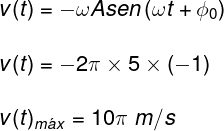Sound and Oscillation

# simple harmonic motion

Because of its simplicity, simple harmonic motion is a physical model that can be used to investigate many more complex systems where there are restorative forces, such as the electrical interactions of atoms and the gravitational attractions between planets.

The MHS is the result of the action of a force that tends to keep some particle, or system of particles, in an equilibrium position , as happens with a spring when stretched or compressed, which is subjected to the action of an elastic force . In this type of movement, the sum of kinetic energy and potential energy is always constant, so we say that there is conservation of mechanical energy.

We call frequency the number of oscillations performed by a system in MHS that are completed every second. The period, in turn, is calculated as the inverse of the frequency and is equal to the time taken for the system in MHS to complete an oscillation. The units of measurement of frequency and period of the MHS are, respectively, the hertz (Hz) and the second (s). The formulas used to calculate these quantities are as follows: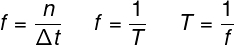f – frequency (Hz)

T – period (s)

n – number of oscillations

Δt – time interval (s)

In addition to the frequency and period quantities, the MHS is defined from angular quantities . Such quantities allow us to know in which position a particle in the MHS is, as well as to specify its measurements of kinetic and potential energy at that instant. The most important of the angular quantities related to MHS is angular frequency , also known as angular velocity or pulsation.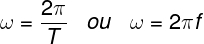The angular frequency has the dimension of rad/s. Radians are one of the different ways to define angles on the trigonometric circle. It is known that one complete turn along the trigonometric circle corresponds to 360º , which in turn correspond to 2π radians .

In the case of MHS, the trigonometric circle serves as a reference for a complete oscillation For example, compressing a spring and releasing it, we have a complete oscillation when it has returned to its initial position – in which case we say that it has traveled an angular displacement equal to 2π radians.

The angular frequency can also be calculated as a function of other parameters, according to the harmonic oscillator type . Among all the possible types of oscillators, the ones that stand out the most for their importance are the simple pendulum and the spring-mass oscillator . The formulas used to calculate the angular frequency in these cases of simple harmonic motion are shown below.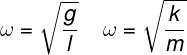## Simple harmonic motion formulas

The most important formulas of simple harmonic motion are the time equations of position, velocity, and acceleration . These equations allow us to determine the position, velocity or acceleration of a mobile in MHS at a given instant of time.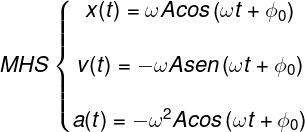In the formulas above, the amplitude (A) is equivalent to the maximum distance that a particle can travel in relation to its equilibrium position The variable t refers to the instant of time, and Φ 0 is called the initial phase and is related to the position at which the system started the movement.

In addition to the formulas already mentioned, there are also formulas that are used to calculate the period of oscillation and the frequency of the simple pendulum and also the mass-spring oscillator, namely: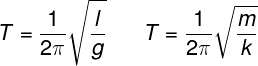## Solved exercises on simple harmonic motion

Question 1 – Calculate the angular frequency of a particle that develops a simple harmonic motion knowing that the period of this motion is equal to 0.5 s.

Template : letter C.

Resolution :

To solve the exercise and calculate the angular frequency of the particle, we need to use the formula that relates this quantity to the period of motion.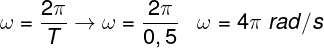According to the calculation made, the angular frequency of the movement is equal to 4π rad/s .

Question 2 – A particle describes a simple harmonic motion of amplitude equal to 4 cm. Knowing that the initial phase of the motion is equal to 0 and that its angular frequency is equal to π rad/s, determine the position of this particle at the instant t = 0.5 s.

a) 2 cm

b) 5 cm

c) 0 cm

d) 4 cm

Template: letter C.

Resolution :

To find out the position of the mobile, it is necessary to use the hourly equation of the position in the MHS; doing so, we must solve the following calculation: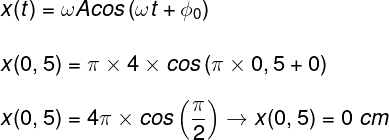Since the cosine of π/2 rad is equal to 0, the result obtained is equal to 0 .

Question 3 – Determine the maximum speed of a mobile that describes a simple harmonic motion of amplitude equal to 5 m knowing that its angular velocity is equal to 2 π rad/s.

a) 2π m/s

b) 10π m/s

c) π m/s

d) π/4 m/s

Template: letter B.

Resolution:

The formula relating the velocity of the particle that develops a simple harmonic motion is shown below. It is noteworthy that, in order to obtain the maximum speed in this type of movement, the sine, on which the speed function depends, must have its value equal to -1; So, just do the following multiplication.